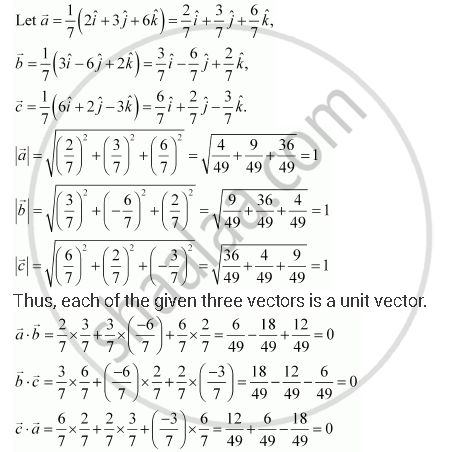Share

# Show that Each of the Given Three Vectors is a Unit Vector:Also, Show that They Are Mutually Perpendicular to Each Other. - CBSE (Science) Class 12 - Mathematics

ConceptProduct of Two Vectors Scalar (Or Dot) Product of Two Vectors

#### Question

Show that each of the given three vectors is a unit vector:

1/7 (2hati + 3hatj + 6hatj), 1/7(3hati - 6hatj + 2hatk), 1/7(6hati + 2hatj - 3hatk)

Also, show that they are mutually perpendicular to each other.

#### SolutionHence, the given three vectors are mutually perpendicular to each other.

Is there an error in this question or solution?

#### Video TutorialsVIEW ALL 

Solution Show that Each of the Given Three Vectors is a Unit Vector:Also, Show that They Are Mutually Perpendicular to Each Other. Concept: Product of Two Vectors - Scalar (Or Dot) Product of Two Vectors.
S